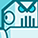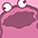# TLE987X BLDC_SENSORLESS_FOC/Current sensing/ CSA gain computationLevel 2
Level 2Hello,
In the "BLDC_SENSORLESS_FOC_LIN_EXAMPLE_TLE987X" SW example, in Emo.c the gain of the CSA is computed as follow:
Could you please tell me to what correspond the value of 1.25 and how it is obtained ? give me please more details
OpGain = 10.0;
CSA_Set_Gain(0u);
/* 1.25 => 125mV, secured OpAmp differential input voltage range */
x = Emo_Focpar_Cfg.NominalCurrent * Emo_Focpar_Cfg.Rshunt * 10.0;

if (x < 1.25)
{
OpGain = 10.0;
CSA_Set_Gain(0u);
}

x = Emo_Focpar_Cfg.NominalCurrent * Emo_Focpar_Cfg.Rshunt * 20.0;

if (x < 1.25)
{
OpGain = 20.0;
CSA_Set_Gain(1u);
}

x = Emo_Focpar_Cfg.NominalCurrent * Emo_Focpar_Cfg.Rshunt * 40.0;

if (x < 1.25)
{
OpGain = 40.0;
CSA_Set_Gain(2u);
}

x = Emo_Focpar_Cfg.NominalCurrent * Emo_Focpar_Cfg.Rshunt * 60.0;

if (x < 1.25)
{
OpGain = 60.0;
CSA_Set_Gain(3u);
}